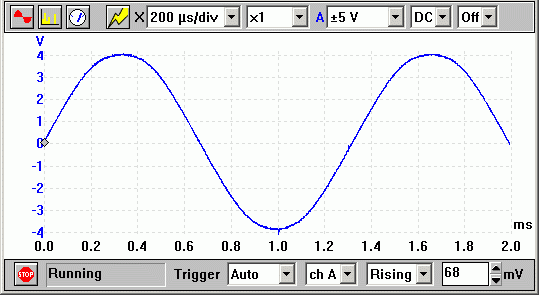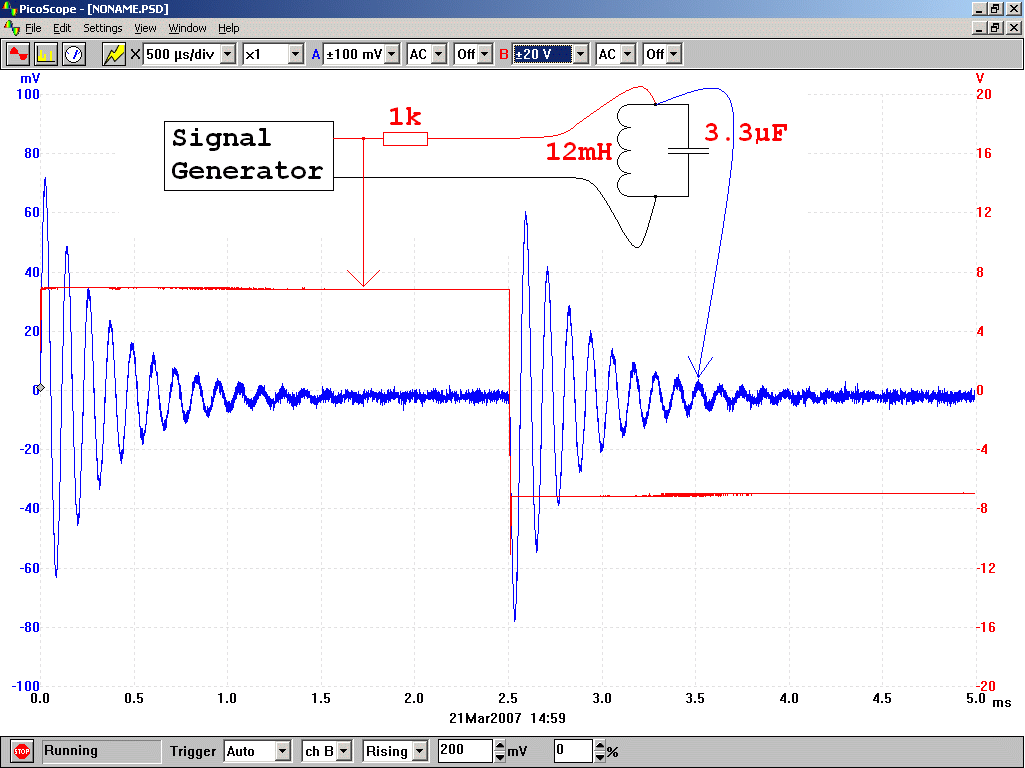RANDOM PAGE

SITE SEARCH

LOG
IN

HELP

# Oscilloscope

This is the AQA version closing after June 2019. Visit the the version for Eduqas instead.

### Measuring Voltage

1. Adjust the Volts per Division setting to its calibrated position.
2. Now set the scale. For example one Volt per division.
3. Now look at the trace and work out how many Volts you are measuring.
4. In the image, it's 4 squares. Each square is worth one Volt so its 4 Volts.### Measuring Period

1. Adjust the Time per Division setting to its calibrated position.
2. Now set the scale. For example 5ms per division.
3. Now look at the trace and work out how many squares each wave takes up.
4. In the image, it's 4 squares. Each square is worth 5ms. So its 4 x 5 = 20ms.### Measuring Frequency

Measure the period and then calculate ...

FREQUENCY = 1 / PERIOD

### Digital Oscilloscopes - Plug into a Computer USB Port

These are generally smaller, cheaper, easier to use and they give more accurate results.Oscilloscopes are used to measure ...

• Peak Voltage
• Peak to Peak Voltage
• RMS Voltage (by calculation        Vrms =   0.7 x Vpeak )
• Time intervals or the period of the wave
• Frequencies by calculating Frequency = 1 / Period
• Wave forms or shapes.

### Sample Oscilloscope QuestionQ: If the Y sensitivity is set to 0.5 Volts per Division, what the the peak voltage? A: The wave is four squares high so the voltage is 4 x 0.5 = 2 Volts Peak.   Q: If the Y sensitivity is set to 2 Volts per Division, what the the peak to peak voltage? A: The wave is eight squares high so the voltage is 8 x 2 = 16 Volts Peak to Peak.   Q: If the Y sensitivity is set to 2 Volts per Division, what the the RMS Voltage? A: The wave is four squares high so the voltage is 4 x 2 = 8 Volts Peak. Multiply by 0.7 to convert to RMS so 8 x 0.7 = 5.6 Volts RMS.   Q: If the X scale is set to 5 ms per division, what the the period of this wave? A: The wave is four squares wide for one complete cycle so the period is 4 x 5 = 20 ms.   Q: If the X scale is set to 5 ms per division, what the the frequency of this wave? A: The wave is four squares wide for one complete cycle so the period is 4 x 5 = 20 ms. Frequency = 1 / Period = 1 / 0.02 = 50 Hz.

### Oscilloscopes have gone digital

Here is a Picoscope trace used to measure the tuned circuit shown in the diagram.• The red square wave is alternating between about +7 Volts and -7 Volts.
• The period of the red square wave is about 5 ms.
• The frequency of the red square wave 1 / Period = 200 Hz.
• The blue damped oscillation has a Period of about 0.11 ms
• The blue damped oscillation has a Frequency of about 9000 Hz.
• The initial amplitude of the blue damped oscillation just over 70 mV.

reviseOmatic V3     Contacts, ©, Cookies, Data Protection and Disclaimers Hosted at linode.com, London